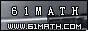Today is . WELCOME: huohai3983/44663  Home | Reg Login | All user | setHomePage | BookmarkHome | Math Test Online | Addition | Subtraction | Multiplication | Division | Time | Worksheet(JPG/PDF) | CAI | Flash Games |Recommendation articleArticle searchHome--maths--Fifth Grade--Inverse relationship of Addition and Subtraction

Inverse relationship of Addition and Subtraction

Inverse relationship of Addition and Subtraction

There is an inverse relationship between addition and subtraction.

If a math fact is considered, for example 3 + 7 = 10. Then the following are also true:

• 10 - 3 = 7
• 10 - 7 = 3

Similar relationships exist for subtraction, for example 10 - 3 = 7. Then the following are also true:

• 3 + 7 = 10
• 7 + 3 = 10

The reason for this is that we are dealing with an equation. An equation is balanced or the same on either side of the equals (=) sign. If exactly the same thing is done to both sides of the equation, it will still be balanced or equal.

In the example above we start with the equation 3 + 7 = 10

• Subtract the same number from both sides 3 + 7 - 3 = 10 - 3
• On the left side the 3 and -3 produce 0 which leaves 7 = 10 - 3
• Turning the equation around to be in more normal form 10 - 3 = 7

#### What number should replace the question marks?

2006-10-24 22:25:28
Viewed 8959 times CloseGreatmathsites